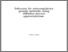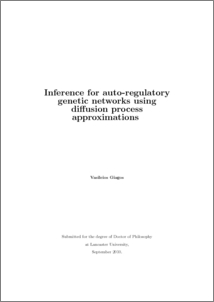# Inference for auto-regulatory genetic networks using diffusion process approximations

Giagos, Vasileios (2010) Inference for auto-regulatory genetic networks using diffusion process approximations. PhD thesis, UNSPECIFIED.Preview
PDF (giagos-thesis)
giagos_thesis.pdf - Accepted Version

## Abstract

The scope of this thesis is to propose new inferential tools, based on diffusion process approximations, for the study of the kinetic parameters in auto-regulatory networks. In the first part of this thesis, we study the applicability of the EA methodology to Stochastic Differential Equations (SDEs) which approximate biological systems. In principle EA can be applied to any scalar-valued SDE as long as a transformation (known as Lamperti transform) exists that sets the (new) infinitesimal variance to unity. We explore the numerical limitations of this requirement by considering a biological system that can be expressed as a scalar non-linear SDE. Next, we consider the multidimensional extension of this transformation and we show, with a counterexample, that EA can be applied to a class of SDEs which is wider than the class of reducible diffusions. In the second part of this thesis, we proposed a reparametrization of the kinetic constants that leads to an approximation known as the Linear Noise approximation (LNA). We prove that LNA converges to a linear SDE, as the size of the biological system increases. Since the LNA is a linear SDE, it has a known transition density with parameters given as the solutions of a system of Ordinary Differential Equations (ODEs) which are usually obtained numerically. Furthermore, we compare the LNA's simulation performance to the performance of other (approximate and exact) methods under different modelling scenarios and we relate the performance of the approximate methods to the system size. In addition, we consider LNA as an inferential tool and we use two methods, the Restarting (RE), which we propose, and the Non-Restarting (NR) method, proposed by Komorowski et. al. (2009) to derive the LNA's likelihood. The two methods differ on the initial conditions that they pose in order to solve the underlying ODEs. We compare the performance of the two methods by considering data generated under different scenarios. Finally, we discuss the lnar, a package for the R statistical environment, that we developed to implement the LNA methodology.

Item Type:
Thesis (PhD)
Departments:
ID Code:
68421
Deposited By:
Deposited On:
24 Jan 2014 06:19
Refereed?:
No
Published?:
Published